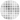# Customize seaborn heatmap

The previous post explains how to make a heatmap from 3 different input formats. This post aims to describe customizations you can make to a heatmap.

You can customize a heatmap in several ways. Following examples will demonstrate these ways.

## Annotate each cell with value

The heatmap can show the exact value behind the color. To add a label to each cell, `annot` parameter of the `heatmap()` function should be set to `True`.

``````# libraries
import seaborn as sns
import pandas as pd
import numpy as np

# Create a dataset
df = pd.DataFrame(np.random.random((10,10)), columns=["a","b","c","d","e","f","g","h","i","j"])

# plot a heatmap with annotation
sns.heatmap(df, annot=True, annot_kws={"size": 7})``````
``<AxesSubplot:>``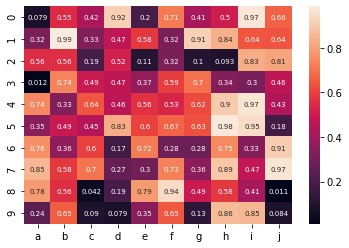## Custom grid lines

The following parameters will make customizations to the heatmap plot:

• `linewidth` : the thickness of the lines
• `linecolor` : the color of the lines
``````# libraries
import seaborn as sns
import pandas as pd
import numpy as np

# Create a dataset
df = pd.DataFrame(np.random.random((10,10)), columns=["a","b","c","d","e","f","g","h","i","j"])

# plot a heatmap with custom grid lines
sns.heatmap(df, linewidths=2, linecolor='yellow')``````
``<AxesSubplot:>``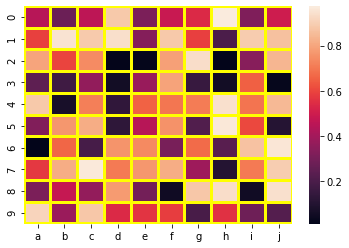## Remove X or Y labels

`yticklabels` and `xticklabels` control the presence / abscence of labels for the Y and X axis respectively.

``````# libraries
import seaborn as sns
import pandas as pd
import numpy as np

# Create a dataset
df = pd.DataFrame(np.random.random((10,10)), columns=["a","b","c","d","e","f","g","h","i","j"])

# plot a heatmap
sns.heatmap(df, yticklabels=False)``````
``<AxesSubplot:>``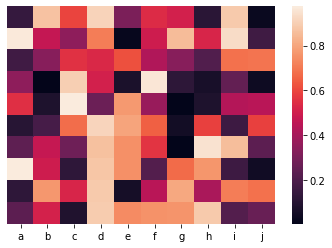## Remove color bar

You can remove the color bar from a heatmap plot by giving False to the parameter `cbar`.

``````# libraries
import seaborn as sns
import pandas as pd
import numpy as np

# Create a dataset
df = pd.DataFrame(np.random.random((10,10)), columns=["a","b","c","d","e","f","g","h","i","j"])

# plot a heatmap
sns.heatmap(df, cbar=False) ``````
``<AxesSubplot:>``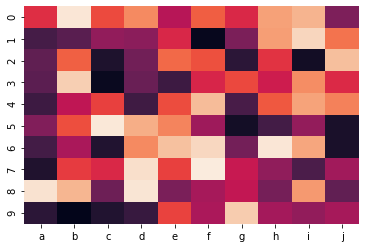## Hide a few axis labels to avoid overlapping

As you can remove x or y labels by setting `xticklabels` or `yticklabels` as False, you can also give an integer to plot only every n label.

``````# libraries
import seaborn as sns
import pandas as pd
import numpy as np

# Create a dataset
df = pd.DataFrame(np.random.random((10,10)), columns=["a","b","c","d","e","f","g","h","i","j"])

# plot a heatmap
sns.heatmap(df, xticklabels=4)``````
``<AxesSubplot:>``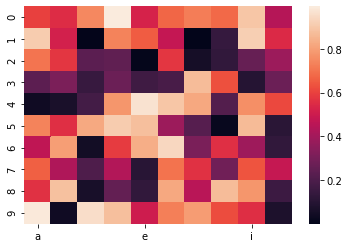## Contact & Edit

👋 This document is a work by Yan Holtz. Any feedback is highly encouraged. You can fill an issue on Github, drop me a message onTwitter, or send an email pasting `yan.holtz.data` with `gmail.com`.